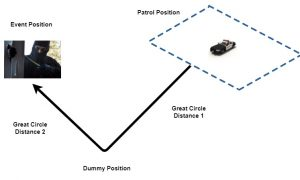In Part II, we added a queue and a PatrolCar class to our Python simulation to answer call events in our police patrol simulation. In this installment, we’ll add calls to our simulation, and do some geographic information system (GIS) distance calculations.

Previously we’d gone over adding latitudes and longitudes in a position to track locations of our patrol units. Now we’re going to introduce some math for distance calculations between two positions that use latitude and longitude. Our first calculating method will be the law of cosines for calculating great circle distance on the surface of a sphere. The low of cosines for great circle distance g is:

g = arccos(sin(lat1)sin(lat2) + cos(lat1)cos(lat2)cos(lon2-lon1))*R

where lat1,lon1 is latitude and longitude for the first position, and lat2, lon2 is latitude and longitude for the second position, and R is the radius of the Earth in miles. R can be in kilometers too, and will give you the great circle distance in kilometers if you use it here. Latitude and longitude must be converted from degrees to radians to use this, and we’ll cover that here. To do all this in or simulation, we need to add this to the top of our Python script:

`import math`

which will import Python’s math library. Unfortunately, now things get complicated. First we’ll need a function to convert degrees to radians, it’s actually not too bad. Here it is:

```#function degrees to radians
return (deg * math.pi / 180)```

So we’re going to pass it a degree measurement, and it’s going to convert it to radians in our great circle calculations, not too complicated. Here’s our great circle distance function in Python:

```def gcd_slc(long1, lat1, long2, lat2):
R = 3959  # Earth mean radius [miles]
d = math.acos(math.sin(lat1) * math.sin(lat2) + math.cos(lat1) * math.cos(lat2) *
math.cos(long2 - long1)) * R
return (d)  # Distance in miles```

It’s pretty much identical to the formula I showed you above, but it incorporates the deg2rad function for degree conversion, and we have to call the math library to access the trigonometric functions in Python.

There’s one more thing I want to incorporate here, when we’re calculating distance, and that’s the notion of Manhattan distance. Manhattan distance is also known as taxicab geometry, rectangular distance, and a few other names, but it’s basically the distance that you have to travel in a region where the roads predominately run north and south, or east and west. In an ideal world we’d incorporate all the roads and features of the  area we’re covering, but we don’t have months to do this, so we’ll just use Manhattan distance. For our area (Phoenix) it’s sufficient. If you working with data in Boston, which was engineered by the Mad Hatter’s association, then it’s not going to work. In fact if you’re working with Boston data then almost nothing is going to work, but let’s not worry about that for now.

All we have to worry about for our Manhattan distance, is placing a “dummy” point between our two positions, that will have the latitude of one of the positions, and the longitude of the other position. Then we can use our great circle function above to go from our first position to our dummy position, then go from our dummy position to our final position.Here’s how it’s implemented in Python:

```def manhattan_dist(latlon1, latlon2):
v_dist=gcd_slc(latlon1.lon,latlon1.lat,latlon1.lon,latlon2.lat)
h_dist=gcd_slc(latlon1.lon,latlon2.lat, latlon2.lon, latlon2.lat)
return(v_dist+h_dist)```

Finally, we’re going to make a Call class, to hold the data objects that we need

```class Call(object):
"""The call process (each call has a ``name``) calls the district
and requests a patrol car.
"""
def __init__(self, name, call_length, latlon, historic_response_time):
self.name=name
self.call_length=call_length
self.latlon=latlon
self.call_log=None
self.historic_response_time=historic_response_time
self.response_time=None```

A lot of the attributes of the class aren’t going to be used right now, the most important thing you need in the Call class is the latlon object, which we’ll use to position the call event. We’ll place the event at minute 13 of our prototype simulation script, and it will look something like this:

```if i == 13:
call_1 = Call(1, 23, LatLon(33.51305,-112.0856),25)
print("Call %d called in at %s %s %s " % (call_1.name,
current_day_hour_minute.day,
current_day_hour_minute.hour,
current_day_hour_minute.minute))
dist1=manhattan_dist(call_1.latlon, car_one.current_latlon)
dist2=manhattan_dist(call_1.latlon, car_two.current_latlon)
if dist1 <= dist2:
print("Car one is closer at distance %.2f miles versus %.2f miles" %(dist1, dist2))
else:
print("Car two is closer at distance %.2f miles versus %.2f miles" % (dist2, dist1))```

So the call is made 13 minutes into the simulation, and we’re going to determine which of the patrol units is closer so we can dispatch it to the call. Next time, we’ll look at populating our simulation with real world events via text file. Here’s the entire script for this tutorial:

https://github.com/jenholm/MontePy/blob/master/Sim5.py